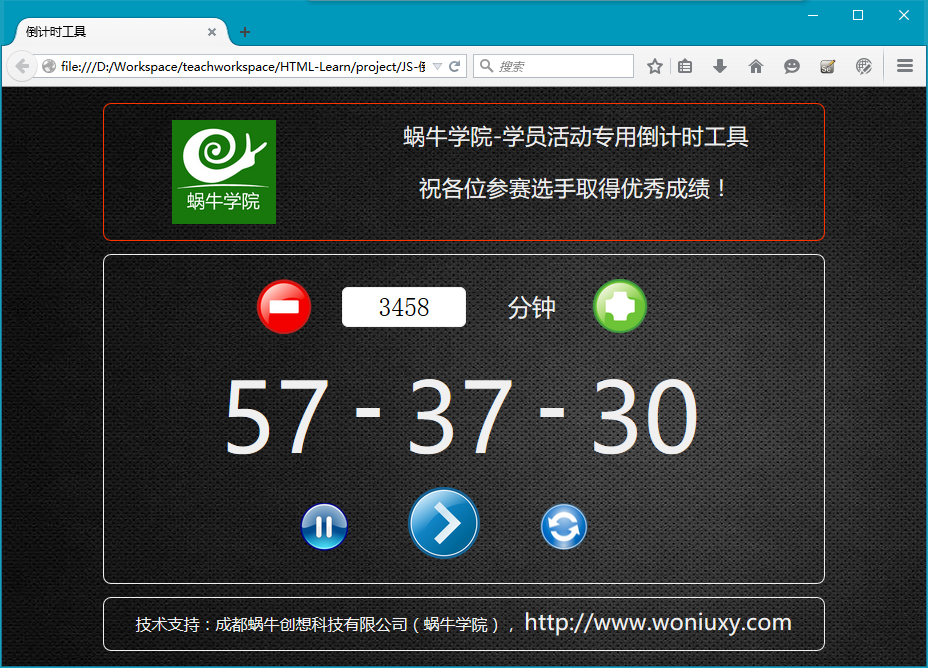# 项目介绍

• 可以自己设置时间，单位为分钟。可以通过两边的加减按钮来微调时间，也可以手工在文本框中输入。

• 倒计时按照“时：分：秒”的方式显示。

• 可以随时暂停计时。

• 当倒计时完成后，自动播放一首音乐用作提醒。# 代码实现

 倒计时工具

00
-
00
-
00

 // 增加1分钟，到最大值5999function plus() {var total = parseInt(document.getElementById("total").value);if (total > 5998)document.getElementById("total").value = 5999;else        document.getElementById("total").value = total + 1;}// 减少1分钟，到最小值1function minus() {var total = parseInt(document.getElementById("total").value);if (total < 2)document.getElementById("total").value = 1;else        document.getElementById("total").value = total - 1;}

 // 检查用户的输入类型，只允许输入1~4位数字function check() {var total = document.getElementById("total");var input = total.value;var pattern = new RegExp("^\\d{1,4}\$");if (!pattern.test(input)) {        total.value = input.substring(0, input.length-1);}}



 function start() {isStarted = true;   //指示已经开始    var totalMinute = parseInt(document.getElementById("total").value);// 如果isPaused的值false，说明没有暂停过，则是全新的倒计时开始    if (isPaused == false)totalSecond = totalMinute * 60;// 开始调用定时器对象完成倒计时（按秒为单位）    interval = setInterval("timerDown()", 1000);}// 倒计时核心程序function timerDown() {// 如果倒计时已经完成，则暂停且播放音乐    if (totalSecond <= 0) {clearInterval(interval);document.getElementById('done').play();}// 如果时分秒等数字只有一位时，前面补0    var hour = Math.floor(totalSecond/3600);if (hour < 10)hour = "0" + hour;var minute = Math.floor((totalSecond-hour*3600)/60);if (minute < 10)minute = "0" + minute;var second = totalSecond % 60;if (second < 10)second = "0" + second;document.getElementById("hour").innerHTML = hour.toString();document.getElementById("minute").innerHTML = minute.toString();document.getElementById("second").innerHTML = second.toString();totalSecond--;  // 让总秒数减1}

 // 暂停计时function pause() {if (isStarted)isPaused = true;clearInterval(interval);}// 刷新页面，重新载入function refresh() {window.location.reload();}

# 思维拓展